## #StackBounty: #neural-networks #python #recommender-system #metric Metrics for implicit data in the recommender system with NCF

### Bounty: 100

Which metrics for analysis and evaluation for implicit data in a recommender system do you use? And which ones do you use when you are looking for the closest neighbors to make a recommendation?

I’m using the NCF model.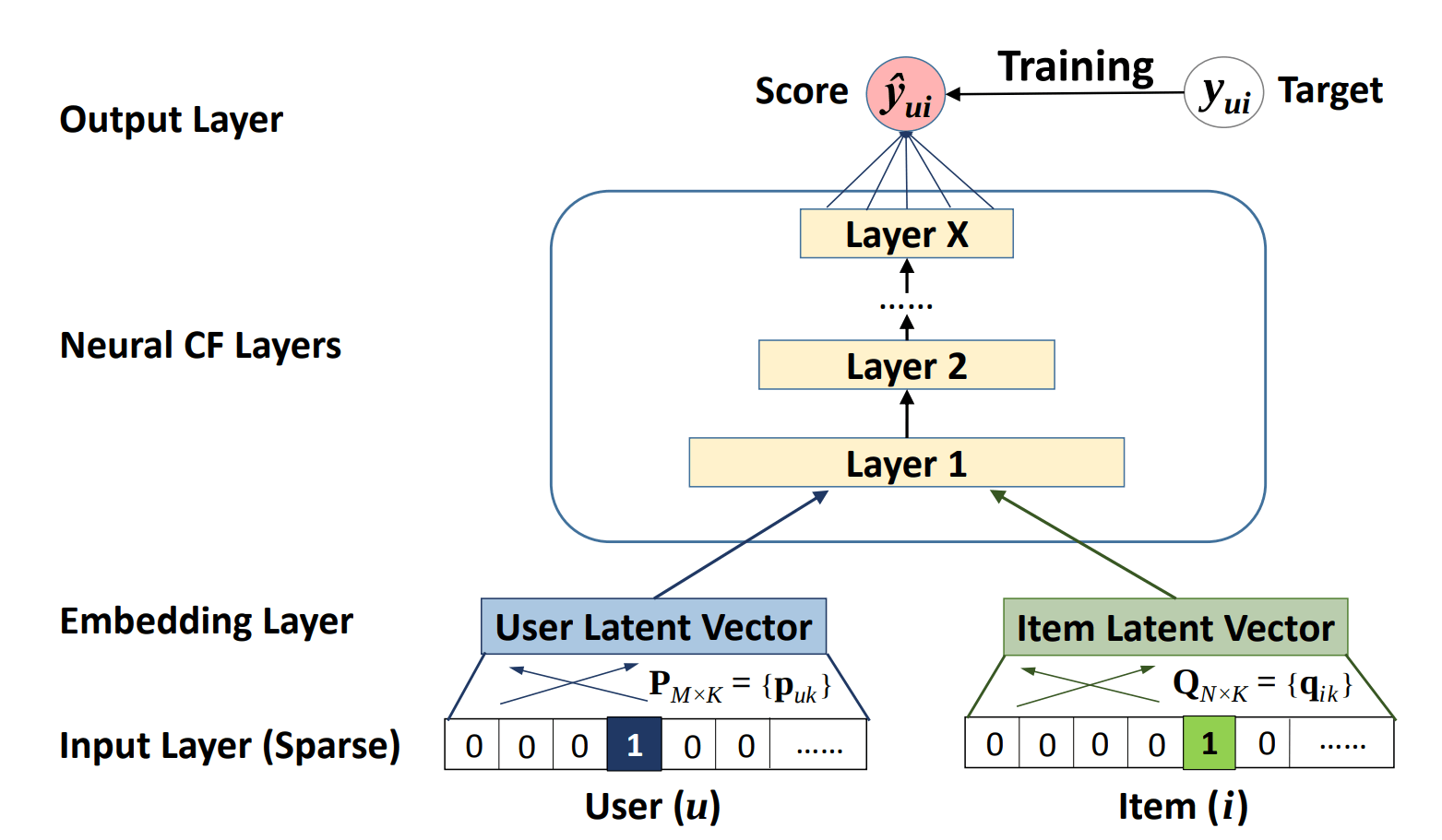The architecture of a Neural Collaborative Filtering model. Taken from the Neural Collaborative Filtering paper.

First I let the model train with the help of the NCF model. Then I find the closest neighbors with k-means.

I found metrics like MSE, RMSE, Precision, Recall, … and What metric should I use for assessing Implicit matrix factorization recommender with ALS? .
I’m not sure which ones are best and how I can then determine whether the closest neighbors are good or bad.

• What metrics are there to evaluate the model?
• What metrics are there to evaluate whether the neighbors found are "good"?

Get this bounty!!!

## #StackBounty: #machine-learning #time-series #neural-networks #python #predictive-models Time series prediction completely off using ESN

### Bounty: 50

I am attempting to predict closing prices based on closing prices extracted from OHLC data from a two-month window with 10 minute intervals (roughly 8600 data points). For this attempt, I am building an echo-state network (ESM), following this tutorial.

With the code below, the prediction is fairly worthless. It looks like noise around an arbitrary average, which does not even resemble the latest data point in the training data. This is nothing close to what the ESN in the tutorial managed at this point. I have tried to alter the results by manually tweaking the hyperparameters `n_reservoir`, `sparsity`, and `spectral_radius`, but all to no avail. During a 4-week course last spring ESNs were briefly touched upon, but not enough for me to understand where I am at fault.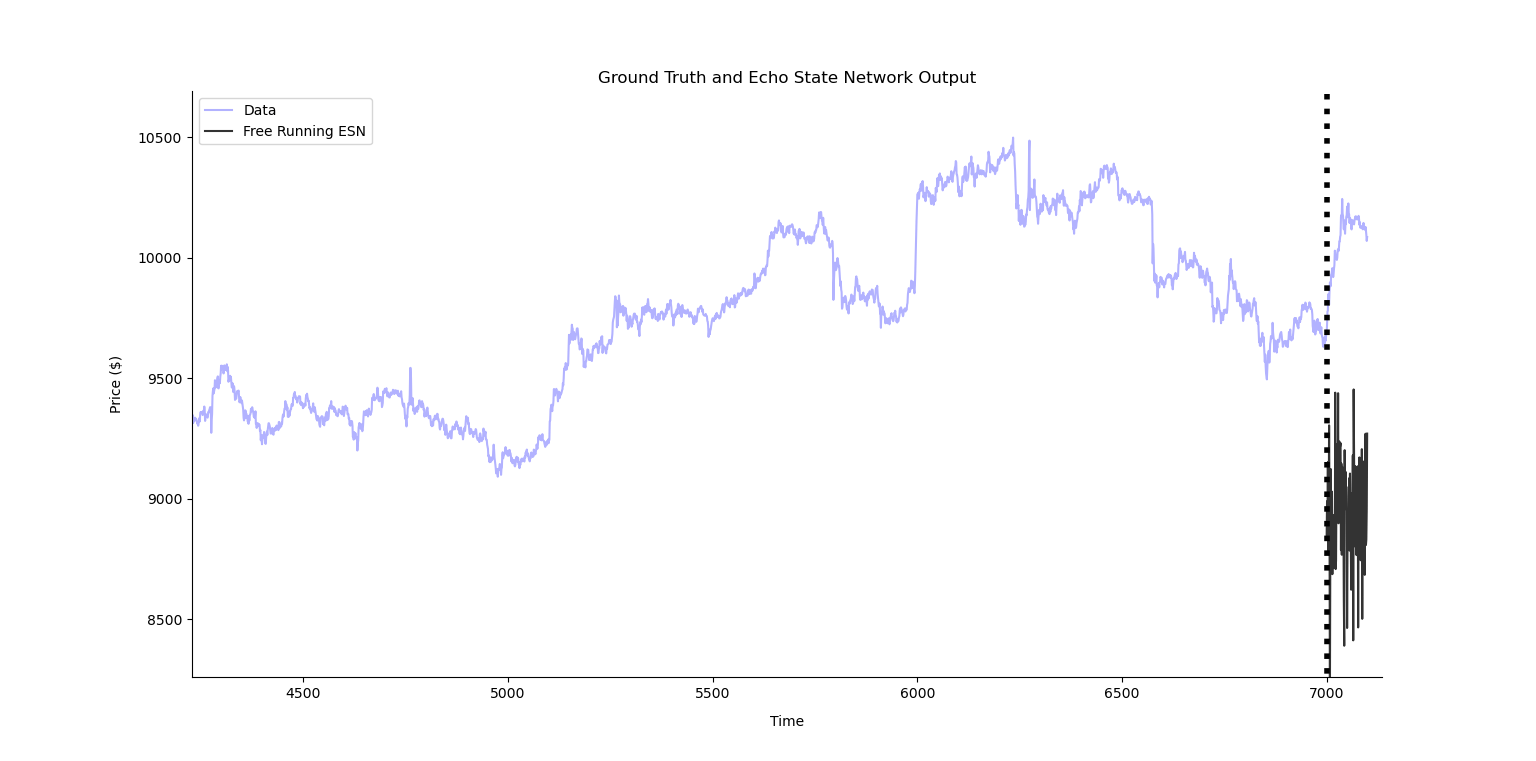My code:

``````import numpy as np
import pandas as pd
import seaborn as sns
from matplotlib import pyplot as plt
from pyESN import ESN
import warnings
warnings.filterwarnings('ignore')

data = df['close'].to_numpy()

n_reservoir = 500
sparsity = 0.2
noise = .0005

esn = ESN(n_inputs = 1,
n_outputs = 1,
n_reservoir = n_reservoir,
sparsity=sparsity,
noise=noise)

trainlen = 7000
future = 10
futureTotal = 100
pred_tot = np.zeros(futureTotal)

for i in range(0,futureTotal,future):
pred_training = esn.fit(np.ones(trainlen),data[i:trainlen+i])
prediction = esn.predict(np.ones(future))
pred_tot[i:i+future] = prediction[:,0]

plt.plot(range(0,trainlen+futureTotal),data[0:trainlen+futureTotal],'b',label="Data", alpha=0.3)
plt.plot(range(trainlen,trainlen+futureTotal),pred_tot,'k',  alpha=0.8, label='Free Running ESN')

lo,hi = plt.ylim()
plt.plot([trainlen,trainlen],[lo+np.spacing(1),hi-np.spacing(1)],'k:', linewidth=4)

plt.title(r'Ground Truth and Echo State Network Output')
plt.legend(loc='best')
sns.despine()
plt.show()
``````

Get this bounty!!!

## #StackBounty: #machine-learning #neural-networks #tensorflow #keras #differential-equations On solving ode/pde with Neural Networks

### Bounty: 50

Recently, I watched this video on YouTube on the solution of ode/pde with neural network and it motivated me to write a short code in Keras. Also, I believe the video is referencing this paper found here.

I selected an example ode
$$frac{partial^2 x(t)}{partial t^2} + 14 frac{partial x(t)}{partial t} + 49x(t) = 0$$

with initial conditions
$$x(0) = 0, frac{partial x(t)}{partial t}rvert_{t=0} = -3$$

According to the video, if I understand correctly, we let the neural network $$hat{x}(t)$$, be the solution of our ode, so $$x(t) approx hat{x}(t)$$

Then, we minimize the ode which is our custom cost function per say. Since, we have initial conditions, I created a step function for individual data point loss:

At, $$t=0$$:
$$loss_i = left( frac{partial^2 hat{x}(t_i)}{partial t^2} + 14 frac{partial hat{x}(t_i)}{partial t} + 49hat{x}(t_i) right)^2 + left( frac{partial hat{x}(t_i)}{partial t} + 3 right)^2 + left( hat{x}(t_i) right)^2$$

else
$$loss_i = left( frac{partial^2 hat{x}(t_i)}{partial t^2} + 14 frac{partial hat{x}(t_i)}{partial t} + 49hat{x}(t_i) right)^2$$

Then, minimize batch loss
$$min frac{1}{b} sum_{i}^{b} loss_i$$

where $$b$$ is the batch size in training.

Unfortunately, the network always learns zero. On good evidence, the first and second derivatives are very small – and the $$x$$ coefficient is very large i.e.: $$49$$, so the network learns that zero output is a good minimization.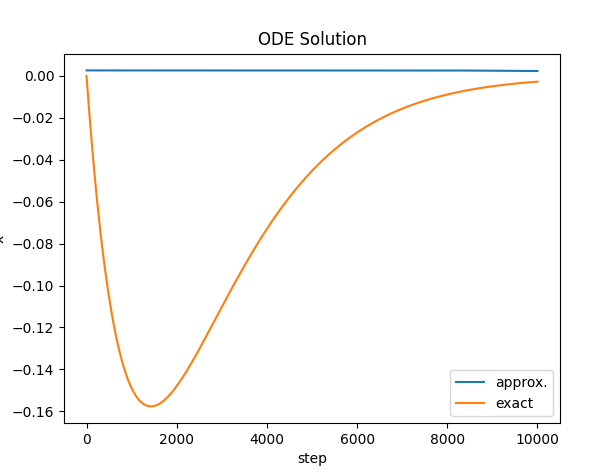Now there is a chance that I misinterpret the video because I think my code is correct. If someone can shed some light I will truly appreciate it.

Is my cost function correct? Do I need some other transformation?

Update:

I managed to improve the training by removing the conditional cost function. What was happening was that the the conditions were very infrequent – so the network was not adjusting enough for the initial conditions.

By changing the cost function to the following, now the network has to satisfy the initial condition on every step:

$$loss_i = left( frac{partial^2 hat{x}(t_i)}{partial t^2} + 14 frac{partial hat{x}(t_i)}{partial t} + 49hat{x}(t_i) right)^2 + left( frac{partial hat{x}(t=0)}{partial t}rvert_{t=0} + 3 right)^2 + left( hat{x}(t=0)rvert_{t=0} right)^2$$

The results are not perfect but better. I have not managed to get the loss almost zero. Deep networks have not worked at all, only shallow one with sigmoid and lots of epochs.

I am surprised this works at all since the cost function depends on derivatives of non-trainable parameters.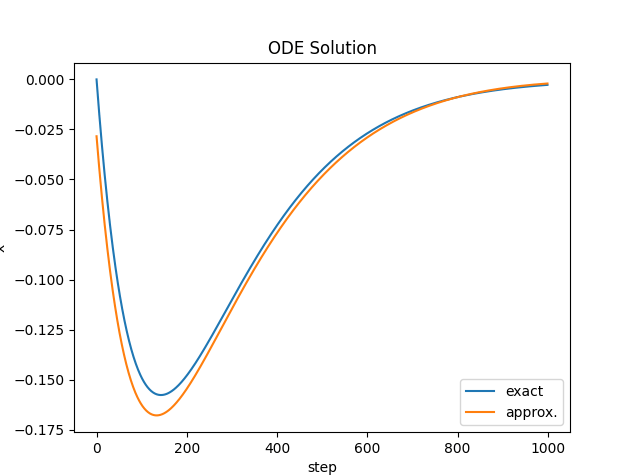I would appreciate any input on improving the solution. I have seen a lot of fancy methods but this is the most straight forward. For example, in the referenced paper above – the author uses a trial solution. I do not understand how that works at all.

Get this bounty!!!

## #StackBounty: #neural-networks #feature-selection #dimensionality-reduction #autoencoders #attention Does Attention Help with standard …

### Bounty: 50

I understand the use of attention mechanisms in the encoder-decoder for sequence-to-sequence problem such as a language translator.

I am just trying to figure out whether it is possible to use attention mechanisms with standard auto-encoders for feature extraction where the goal is to compress the data into a latent vector?

Suppose we had a time series data with N dimensions and we wanted to use an auto-encoder with attention mechanisms (I am thinking of a self-attention because I think it is more appropriate in this case – I might be wrong) to better learn interdependence among the input sequence and thus we would get a better latent vector L.

Or it could be better to use Recurrent Neural Network or its variants in this case.

Does anyone have better thoughts or an intuition behind this?

Get this bounty!!!

## #StackBounty: #neural-networks #keras #torch he_normal (Keras) is truncated when kaiming_normal_ (pytorch) is not

### Bounty: 50

Thanks for having a look at my post.

I had an extensive look at the difference in weight initialization between pytorch
and Keras, and it appears that the definition of he_normal (Keras)
and kaiming_normal_ (pytorch) is different across the two platforms.

They both claim to be applying the solution presented in He et al. 2015 (https://arxiv.org/abs/1502.01852) :
https://pytorch.org/docs/stable/nn.init.html,
https://www.tensorflow.org/api_docs/python/tf/keras/initializers/HeNormal.
However, I found no trace of truncation in that later paper.
To me truncation makes a lot of sense.

Do I have a bug in my simple code that follows, or indeed these two platforms claim
to apply a solution from a paper, but differ in their implementation.
Then how is correct? What is best?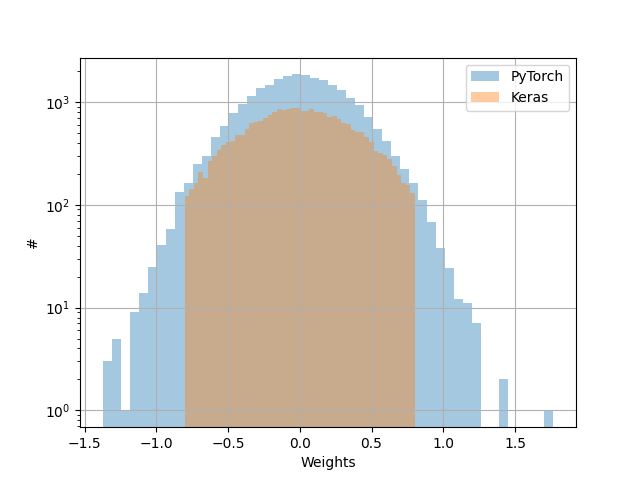``````import numpy as np
import matplotlib.pyplot as plt

import torch

import keras
import keras.models as Model
from keras.layers import Input
from keras.layers.core import Dense

real = 100

### pyTorch
params = np.array([])
for _ in range(real):
lin = torch.nn.Linear(in_features=16, out_features=16)
torch.nn.init.kaiming_normal_(lin.weight)
params = np.append(params,lin.weight.detach().numpy())
params = params.flatten()
plt.hist(params,bins=50,alpha=0.4,label=r'PyTorch')

### Keras
params = np.array([])
for _ in range(real):
X_input = Input()
X = Dense(units=16, activation='relu', kernel_initializer='he_normal')(X_input)
model = Model.Model(inputs=X_input,outputs=X)
params = np.append(params,model.get_weights())
params = params.flatten()
plt.hist(params,bins=50,alpha=0.4,label=r'Keras')

###
plt.xlabel(r'Weights')
plt.ylabel(r'#')
plt.yscale('log')
plt.legend()
plt.grid()
plt.show()
``````

Get this bounty!!!

## #StackBounty: #neural-networks #dataset #sample Is it better to split sequences into overlapping or non-overlapping training samples?

### Bounty: 50

I have $$N$$ (time) sequences of data with length $$2048$$. Each of these sequences correseponds to a different target output. However, I know that only a small part of the sequence is needed to actually predict this target output, say a sub-sequence of length $$128$$.

I could split up each of the sequences into $$16$$ partitions of $$128$$, so that I end up with $$16N$$ training smaples. However, I could drastically increase the number of training samples if I use a sliding window instead: there are $$2048-128 = 1920$$ unique sub-sequences of length $$128$$ that preserve the time series. That means I could in fact generate $$1920N$$ unique training samples, even though most of the input is overlapping.

I could also use a larger increment between individual "windows", which would reduce the number of sub-sequences but it could remove any autocorrelation between them.

Is it better to split my data into $$16N$$ non-overlapping sub-sequences or $$1920N$$ partially overlapping sub-sequences?

Get this bounty!!!

## #StackBounty: #neural-networks #conv-neural-network #tensorflow #invariance High resolution in style transfer

### Bounty: 100

I’m investigating a bit about neural style transfer and its practical applications and I’ve encountered a major issue. Are there methods for high resolution style transfer? I mean, the original Gatys’ algorithm based on optimization is obviously capable of producing high resolution results, but it’s a slow process so it’s not valid for practical use.

What I’ve seen is that all pretrained neural style transfer models are trained with low-resolution images. For example, tensorflow example is trained with 256×256 style images and 384×384 content images. The example explains that the size of the content can be arbitrary, but if you use 720×720 images or higher, the quality drops a lot, showing only small patterns of the style massively repeated. If you upscale content and style size accordingly, the result is even worse, it vanishes. Here are some examples of what I’m explaining:The original 384×384 result with 250×250 style size1080×1080 result with 250×250 style size. Notice that it just repeats a lot those small yellow circles.1080×1080 result with 700×700 style size. Awful result.

So my question is, is there a way to train any these models with size invariance? I don’t care if I have to train the model myself, but I don’t know how to get good, fast and arbitrary results with size invariance.

Get this bounty!!!

## #StackBounty: #machine-learning #hypothesis-testing #neural-networks #statistical-significance #multiple-comparisons Comparing differen…

### Bounty: 50

Say, I have an image dataset (for example, imagenet) and I am training two image recognition models on it.
I train a resnet with 10 layers 3 times on it (each time with different random weight initialization), each time for 20 epochs. For last 5 epochs of training, the accuracy on test datasets does not change very much, but oscillates around. At each of the last 5 epochs, I save the current weights (at that epoch) of the model.

I also have a resnet with 20 layers. Let’s say I train it 4 times for 20 epochs on the same dataset, and simiarly save the weights at the final 5 epochs for each training.

I also have 10 test image datasets, coming from various sources, maybe from internet, web cameras, street cameras, screenshots from movies, etc.
Each of the the datasets has varying number of images in them, ranging from 20 to 20000.

I evaluate all the models (2(3+4)5=70) on all the datasets.

Now given the above info, I have these questions:
What is the probability that a resnet with 20 layers is on average better on these datasets than a resnet with 10 layers? (on average, as in calculating the accuracy on each of the ten datasets, and then taking the mean of the ten resultant values). And what are the confidence intervals (or credible intervals) around that probability value?

There are multiple sources of variance here: variance due to test dataset sizes, variance due to different weight initializations, variance due to accuracy oscillating from one epoch to next. How do you account for all these sources of variance to come up with a single number which would indicate the probability that one method is better than the other?

And finally, imagine that you did these tests, and you noticed that on one of the ten datasets the accuracy difference is the largest between these two methods. How can you quantify whether such accuracy difference is by chance or because it indeed is the case that one of the methods is better on this particular dataset? (the concern here is the multiple hypothesis testing and how to account for it, while taking care of all the other sources of variance as well).

Get this bounty!!!

## #StackBounty: #neural-networks #conv-neural-network How to add appropriate noise to a neural network with constant weights so that back…

### Bounty: 50

I have a neural network in a synthetic experiment I am doing where scale matters and I do not wish to remove it & where my initial network is initialized with a prior that is non-zero and equal everywhere.

How do I add noise appropriately so that it trains well with the gradient descent rule?

$$w^{} := w^{} – eta nabla_W L(W^{})$$

cross-posted:

Get this bounty!!!

## #StackBounty: #neural-networks #reinforcement-learning #tensorflow #q-learning As epsilon decays, rewards gets worse during exploitatio…

### Bounty: 50

I am currently trying to write learning agent from the "Human Level Control in DRL" Paper in Tensorflow 2.0. I’ve copied the recommended hyperparameters and picked the easiest environment possible. There has to be an error in my model because the rewards decrease the more epsilon decreases.

My model is identical to the one described in the paper (at least, I think it is).

``````def build_model(self, agent_history_length):
# 4 greyscaled 84*84 images as an input
inputs = keras.Input(shape=(agent_history_length, 84, 84))
l1 = keras.layers.Conv2D(
filters=32,
kernel_size=(8, 8),
strides=(4, 4),
activation=keras.activations.relu,
name="cnn_1",
)(inputs)
l2 = keras.layers.Conv2D(
filters=64,
kernel_size=(4, 4),
strides=(2, 2),
activation=keras.activations.relu,
name="cnn_2",
)(l1)
l3 = keras.layers.Conv2D(
filters=64,
kernel_size=(3, 3),
# stride=(1, 1),
activation=keras.activations.relu,
name="cnn_3",
)(l2)
flatten = keras.layers.Flatten()(l3)
l4 = keras.layers.Dense(
512,
activation=keras.activations.relu,
name="dense_1"
)(flatten)
# for every action, there is an output
outputs = keras.layers.Dense(
self.num_actions,
activation=keras.activations.relu,
name="output"
)(l4)
optimizer = keras.optimizers.RMSprop(learning_rate=self.learning_rate, rho=.99, momentum=.95)
model = keras.Model(inputs=inputs, outputs=outputs)
self.model = model
# use rmsprop (as used in Human-level control through deep
# reinforcement learning)
self.model.compile(optimizer=optimizer, loss=tf.keras.losses.MeanSquaredError())
``````

My training function should be the same as described in the paper too.

``````def learn(self):
# retrieve 32 samples
s, a, r, s_, d = self.experience_memory.pop()
if s is None:
return

q_vals = self.network.model.predict(s)

# retrieve the next expected maximum reward (from target network)
q_vals_next = self.target_network.model.predict(s_)

# y_values
q_target = q_vals_next.copy()

batch_index = [x for x in range(batch_size)]

# bellman equation
q_target[batch_index, a] = r + gamma * np.max(q_vals_next) * (1-d)

self.network.model.fit(s, q_target, verbose=0)

# update the target network every 10 episodes
if self.current_step % (episode_steps * 10) == 0:
self.target_network.model.set_weights(self.network.model.get_weights())
``````

Something is off and I can’t find the cause. I’d be very grateful if someone could point me in the right direction.

Get this bounty!!!